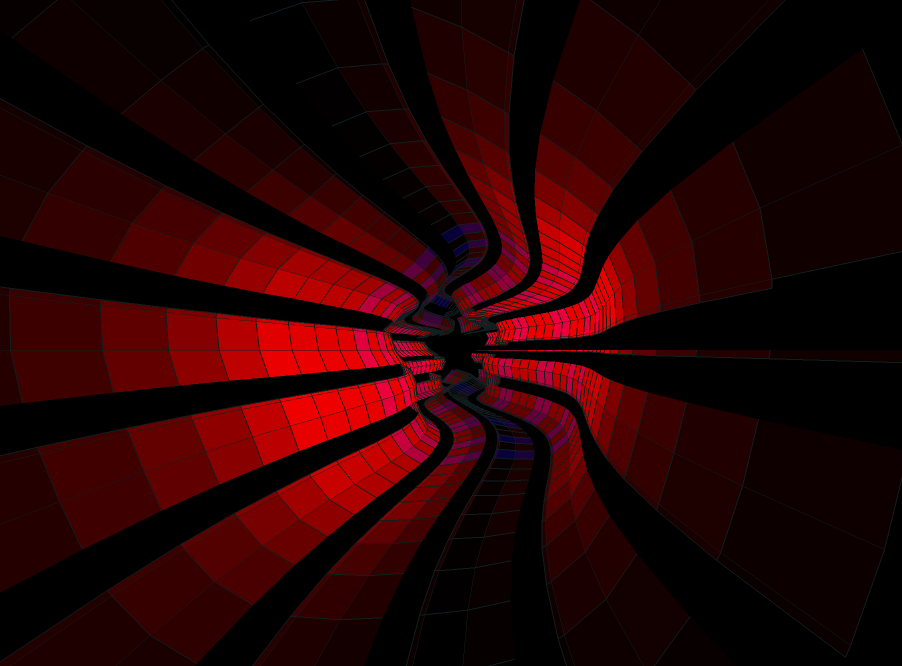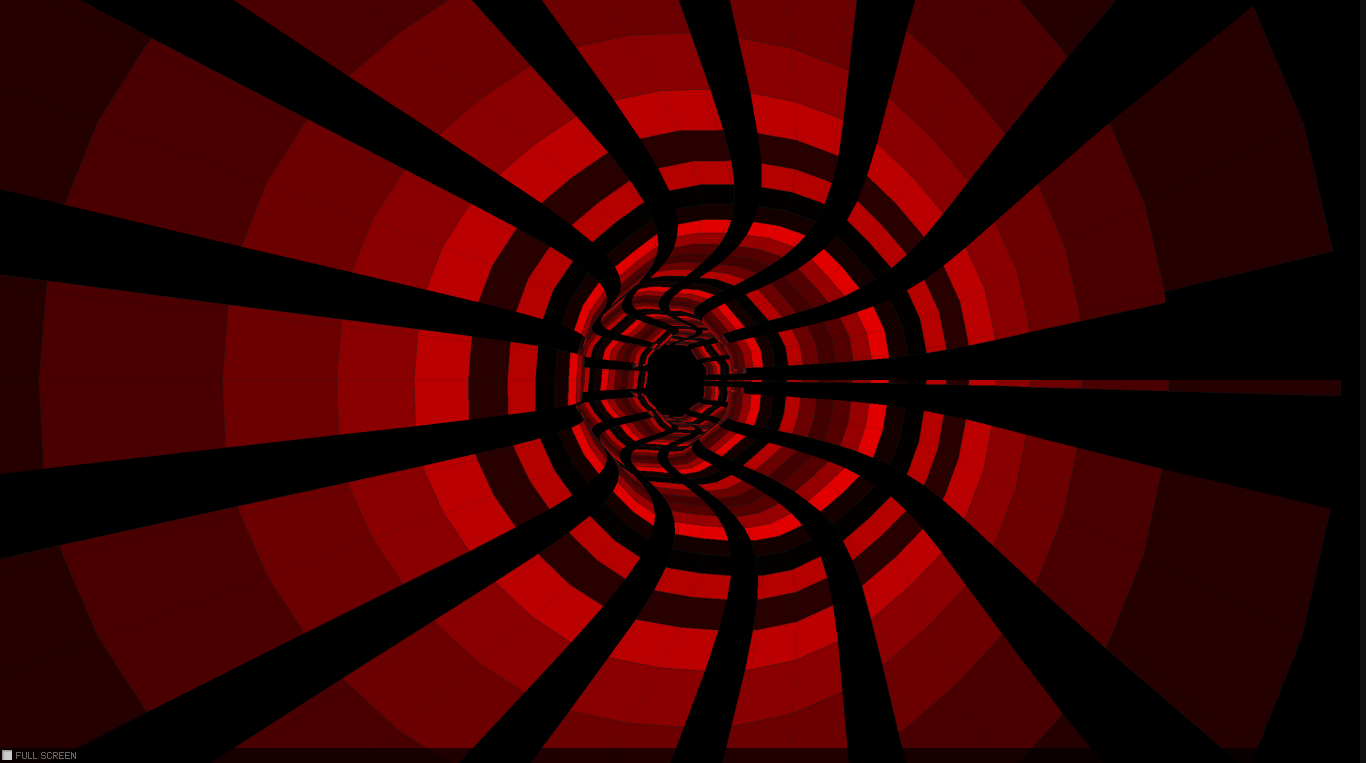﻿ Endre Simo - Sound visualization

# Sound visualization

Categories: Actionscript 3,Blog,Experiments,Source Codes,Tutorials,Visualization

Sound visualization is one thing which I was always fascinated by. I stumbled upon this small Javascript experiment and thought would be nice to reproduce it in Actionscript, adding some spicy extra addons like interaction and sounds reacting shapes. So I decided to implement in Actionscript. Actually it went very well, in fact i only retained the core algorithm. The core algorithm consist in just a few lines:

``````private function createSurface(a:Number, z:Number):Point3D
{
var r:Number = W/10;
var R:Number = W/3;
var b:Number = -10*Math.cos(a*5 + T);

return new Point3D(W/2 + (R*Math.cos(a) + r*Math.sin(z+2*T))/z + Math.cos(a)*b,
H/2 + (R*Math.sin(a))/z + Math.sin(a)*b, Z);
}

private function drawQuad(a:Number, da:Number, z:Number, dz:Number):void
{
var v:Array = [createSurface(a,z),
createSurface(a+da,z),
createSurface(a+da, z+dz),
createSurface(a, z+dz)];

_points = new Vector.();
_stroke = new GraphicsStroke();
_stroke.thickness = 1;
_stroke.fill = new GraphicsSolidFill(0x102020,0.95);

_path = new GraphicsPath(new Vector.(), new Vector.());
_path.moveTo(Point3D(v).x, Point3D(v).y);

for (var i:Number = 0; i < v.length; i++)
{
_path.lineTo(Point3D(v[i]).x, Point3D(v[i]).y);
}
_background = new GraphicsSolidFill(0x663399, 0.95);
}``````

First we create some quads using sine and cosine, multiplying by the radius, which is given as a constant. Then we store these quads on an array, setting their positions by calling the GraphicsPath.lineTo method. These positions are updated each time we loop through on an EnterFrame event listener. The fancy things happens here, where we use a fog variable to apply the shading effect by tweaking some depth illusion.

And this is how it looks after implementation. By the way this is a good example of how robust and fast are <IGraphicsData> and drawGraphicsData functions combined.(Move the mouse to change the color shading intensity)

After implementing the basic structure I thought - following the suggestion of some of the active members of wonderfl community - it would be nice to add some extra feature like sound visualization. Initially this little effect consists of some sinusoidally  curvy movement modifying the color intensity by the mouse position. You can check the effect by clicking on the image above.

I was pleasantly surprised by the smoothly frame rate of the effect, so as i mentioned earlier the next step was to implement the algorithm for sound visualization. Thanks to makc3d audio API it was an easy job to obtain some samples which later could be used for the funky disco effect following the rhythm of the sound. This simple API connects to BeatPort portal, and by providing an unique key we can select from a various music genres. I opted for dubstep only because the high variation of beats can produce a very differentiated transition (a next step could be to implement an interface for selecting the different music channel).

This is another variation.

Playing with the values we can obtain different visualization effects. For example scaling up the bass bit rate we can obtain a nice upscaled flowing ring or reducing the radius value we should obtain a perfect circular cube like in the example below.

Definitely a GUI interface would have been a better choice for playing with values, but because i put together this experiment in almost two hours i didn't take the effort to polish the code. Maybe in a future time.(Scroll the mouse to jump to the next track)

You can grab the source code here!

### Show comments:

comments powered by Disqus